### Home > PC > Chapter 4 > Lesson 4.1.4 > Problem4-53

4-53.
1. For each graph below, find an equation using sine and an equation using cosine that will generate the graph. For each graph, one of the functions you find should not require a horizontal shift. Homework Help ✎

1.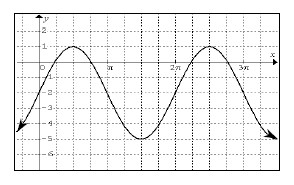2.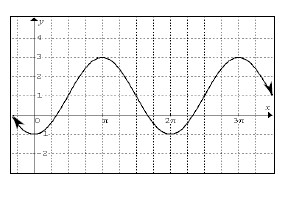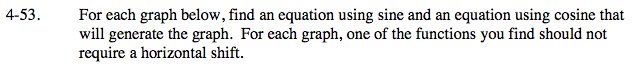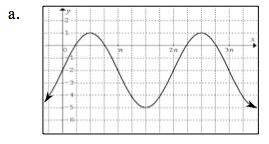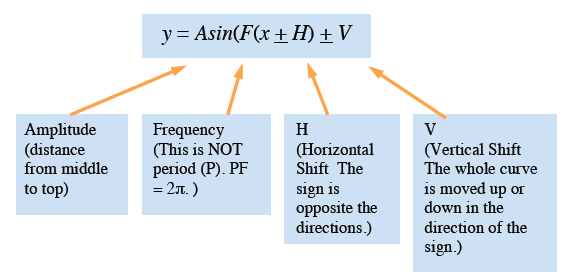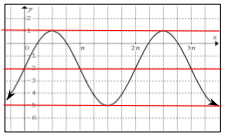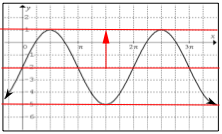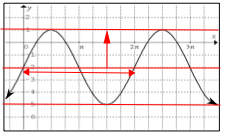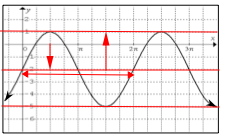Draw a horizontal line through the center, top, and bottom of the curve.

The amplitude is from the middle up to the top of the curve.

The period is the length of one cycle. In this case it is 2π, so F = 1.

Shifts: Horizontal (left or right shift from y-axis). Vertical (up or down shifts from x-axis.)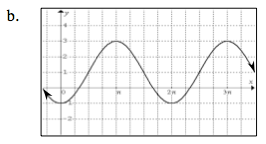Use the eTool below to visualize this problem.
Click on the link to the right to view the full version of the eTool: PCT 4-53 eTool